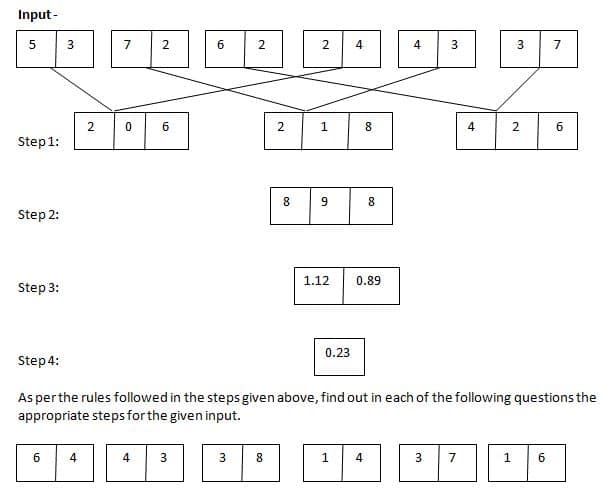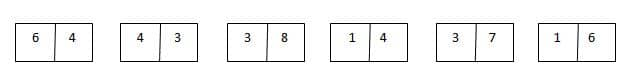# gkrecall

We brings you daily Current affairs, daily Current Affairs Quiz, weekly Current Affairs, weekly Current Affairs Quiz to enhance your preparation for upcoming exams. We also provide articles related to quant, reasoning, English, ssc etc .

## Breaking

We bring you the complete and important daily Reasoning  to achieve more marks in Banking, Insurance, UPSC, SSC, CLAT, Railways and all other competitive Exams. We prepare it based on our daily current affairs.Hope you like it.

START QUIZ
Directions (1 – 5): Study the following information carefully to answer the given questions1. What is the multiplication of all the numbers of step 2?
A. 8
B. 6
C. 12
D. 15
E. None of these
2. Which is the largest number obtained in step 1?
A. 188
B. 244
C. 249
D. 289
E. 250
3. If the third number of all the boxes is halved and then added the half numbers, what is the result in step 1?
A. 10.5
B. 12.5
C. 8.5
D. 11.5
E. None of these
4. What is the sum of numbers in step 3?
A. 0.66
B. 0.74
C. 1.83
D. 1.66
E. 1.35
5. Which is the following number obtained in last step?
A. 1.07
B. 1.77
C. 1.67
D. 1.17
E. None of these
Solution-
In first step:
Multiply 1st and 2nd number of box 1 and 4 respectively: 5 × 4 = 20
Multiply 2nd and 1st number of box 1 and 4 respectively: 3 × 2 = 6
Multiply 1st and 2nd number of box 2 and 5 respectively: 7 × 3 = 21
Multiply 2nd and 1st number of box 2 and 5 respectively: 2 × 4 = 8
Multiply 1st and 2nd number of box 3 and 6 respectively: 6 × 7 = 42
Multiply 2nd and 1st number of box 3 and 6 respectively: 2 × 3 = 6In second step:
Add 1st and 3rd number of box 1 and subtract it from 2nd number of box 1 = 6 + 2 = 8, 8 – 0 = 8
Add 1st and 3rd number of box 2 and subtract it from 2nd number of box 2 = 8 + 2 = 10, 10 – 1 = 9
Add 1st and 3rd number of box 3 and subtract it from 2nd number of box 3 = 6 + 4 = 10, 10 – 2 = 8
In third step:
Divide 2nd number from 1st number = 9 ÷ 8 = 1.12
Divide 3rd number from 2nd number = 8 ÷ 9 = 0.89
In fourth step:
Subtract both the numbers = 1.12 – 0.89 = 0.23In first step:
Multiply 1st and 2nd number of box 1 and 4 respectively: 6 × 4 = 24
Multiply 2nd and 1st number of box 1 and 4 respectively: 4 × 1 = 4
Multiply 1st and 2nd number of box 2 and 5 respectively: 4 × 7 = 28
Multiply 2nd and 1st number of box 2 and 5 respectively: 3 × 3 = 9
Multiply 1st and 2nd number of box 3 and 6 respectively: 3 × 6 = 18
Multiply 2nd and 1st number of box 3 and 6 respectively: 8 × 1 = 8
In second step:
Add 1st and 3rd number of box 1 and subtract it from 2nd number of box 1 = 4 + 2 = 6, 6 – 4 = 2
Add 1st and 3rd number of box 2 and subtract it from 2nd number of box 2 = 9 + 2 = 11, 11 – 1 = 3
Add 1st and 3rd number of box 3 and subtract it from 2nd number of box 3 = 8 + 1 = 9, 9 – 8 = 1
In third step:
Divide 2nd number from 1st number = 3 ÷ 2 = 1.5
Divide 3rd number from 2nd number = 1 ÷ 3 = 0.33
In fourth step:
Subtract both the numbers = 1.5 – 0.33 = 1.17
Direction (6-10): In each question below is given a group of numbers / symbols followed by five combinations of letter codes numbered (1), (2), (3), (4) and (5). You have to find out which of the combinations correctly represents the group of numbers / symbols based on the following coding system and the steps given and mark the number of that combination as your answer:

Steps to be followed as per the given order:
(1) All the numbers are to be sorted in ascending order and then written in the place of numbers only
(2) In the series obtained after the step one if it contains number preceded by symbol than both should interchange
(3) In the series obtained after the step two if odd number is followed by even number then subtract the lower number from higher number to obtain the new series thus formed. The new series which will be formed by following the above steps will be then coded according to the table values given above.
6. What is the code for 72*6@?
A. PEMON
B. PFNON
C. PFMUN
D. PFMON
E. None of these
7. What is the code for 49+>%?
A. GPATW
B. GPAUW
C. GPAUV
D. GQAUW
E. GPOUW
8. What is the code for 86&*4?
A. GFIPO
B. GEIQO
C. GFIQN
D. GFIQO
E. None of these
9. What is the code for +49<@?
A. None of these
B. GAPHN
C. GAPGN
D. GAOHN
E. GAPHJ
10. What is the code for 68\$%2?
A. PECQW
B. PFDQW
C. PFCQV
D. PFCQW
E. None of these
Solution-
6. Option D
7. Option B
8. Option D
9. Option B
10. Option D